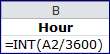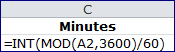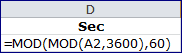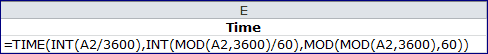Convert Seconds to a Time Format in Excel

The last several posts have shown how to convert and aggregate a given number of seconds that represent song duration. A simple example because song duration never exceeded one hour.

But what if your data has values greater than 3600 seconds? How would you convert those seconds into an hour:minute:second format?

Luckily, the previously mentioned functions: TIME, INT, MOD still work. However, the arguments get a little more complicated.

To simplify things we’ll look individually at the three TIME Function arguments: Hours, Minutes, Seconds. Going so far as to create a formula for each, then combining into one big formula.

A Short Review

The INT Function returns the integer portion of a division and the MOD Function returns the remainder. If I divide 7 by 2, then INT(7/2) = 3 and MOD(7,2) = 1. Two goes into seven, three times, with one left over.

Additionally, there are two conversion factors.

• 1 hour = 60 minutes/hour x 60 seconds/minute = 3600 seconds
• 1 minute = 60 seconds/minute = 60 seconds

The TIME Function Arguments

In the following example, the value in cell A2 is 3661 seconds, which is 1 hour, 1  minute and 1 second.

Hours Argument

Getting the number of hours is easy, just use A2/3600 for the INT Function argument.Minutes Argument

We need the remainder of A2/3600, which is MOD(A2,3600) = 61, but still have to convert these seconds to minutes. The answer is to divide MOD(A2,3600) by 60 and get the integer portion for the number of minutes.In other words, 3600 goes into 3661 once, with 61 seconds left over. The MOD function gives us the 61 seconds. The INT function argument then reduces to INT(61/60), which gives us one (1), because 60 goes into 61 once.

Seconds Argument

MOD(A2/3600) gives us 61 seconds, so we need to divide by 60 and get the remaining seconds by using the MOD function again.Since the inside MOD function equals 61 seconds, MOD(61,60) gives the us one (1), because after you divide 61 by 60 there is one (1) second left.

TIME Function

Putting all of these together inside the TIME Function makes the formula rather large.Yet knowing how the functions work in each argument makes things a little more understandable.

Another TIME Function Example

Since 1:01:01 was easy math, but lousy use of too many 1’s I’ll use the value in cell A3, 7510, as a second example.

• Hours: INT(7510/3600) = 2 hours
• Remainder: MOD(7510, 3600) = 510 seconds
• Minutes: INT(510/60) = 5 minutes
• Seconds: MOD(510,60) = 10 seconds
• TIME(2,5,10) = 2:5:10

Notice the Remainder of the first division (7510/3600) is the central argument for Minutes and Seconds.9 thoughts on “Convert Seconds to a Time Format in Excel”

1.Bob Burg

Hi,
This seems to work only if total time is less than 24 hours. If total seconds is 86,399 or less, it works. If total seconds is 86,400 or greater, it doesn’t seem to work. The Excel ‘Time’ function doesn’t seem to correctly handle a number 24 or greater in the hours bucket. I can calculate it and format it, just can’t use the Excel function. The situation comes up with machine run-time hours. Ideas?

1.Gregory

You can always convert your number to a time serial number and use Excel custom formatting to show the time format correctly. You just have to remember to use square brackets around any cumulative number you want to represent.

As an example, lets say you have a number of seconds like 129600, which is more that one day. To covert to a time serial number, just divide by 86400, which gives you 1.5. Then change the cell formatting to [h]:mm:ss and you will see 36:00:00 which is 36 hours.

Another example. To show 46.5 in an hours and minutes format, just divide by 1440 minutes (the number of minutes in a day) and then change the cell formatting to the same custom format [h]:mm:ss and you will see 0:46:30 or you can use [m]:ss to leave off the hours and show just 46:30.

So the key is to convert to a time serial number by dividing by the lowest common denominator (24 to show hours, 1440 to show minutes, or 86400 to show seconds) and then use a custom time format with [] square brackets that allow cumulative time in the formatting. A time serial number is a fractional number between zero and 1.

1.Bob Burg

Awesome! Just what I needed. It converted 151143.211708 seconds to 41:59:03 perfectly after I divided by 86400 and set the custom time format for the cell. It also worked correctly for small numbers like 50 seconds and 130 seconds. Thanks for your help. For most calculations, I plan to use this instead of the Excel ‘Time’ function to avoid potential unrecognized errors due to the 24-hour limitation of the “Time” function. Other than deciding correctly what to divide by, are there any limitations or caveats using this process?

1.Gregory

Not really, once you decide on a conversion factor it’s easily checked for the right answer. Excel does an excellent job of dealing with a “serial time” value.

2.Joakim Svahn

One limitation seems to be that it works up to 255611462399 seconds, i.e. up to 31 days + 23:59:59.

1.Gregory

31 days + 23:59:59 = 2764799 seconds. The number you’ve given, 255611462399 is so large that if you divide by 86400 (one day of seconds) you get 2958466 days, which is about 8105 years. That’s a lot of seconds.

2.Mara

Hi Gregory… I have a situation where I have total hours (27) and the cell calcs to 3 hours (cause it’s more than 24 hours). How can I convert/calc this to show 27 hours?
It’s driving me nuts – I’ve tried different variations of your samples and nothing!
Hope to hear from you soon.

1.Gregory

Select the cell with the 3:00 and use the keyboard combination Control+1 (or Command+1 on a Mac) to bring up the Format Cells dialog box. Click on the Number tab, and under Category, select Custom where you will see something in the type box like this

h:mm;@

Change it to add square brackets around the h so that Excel will treat hours as a cumulative number, instead of one that repeats every 24 hours. Here’s what I used for your example.

[h]:mm:ss

This will show the 27 hours (plus and minutes and seconds too).